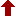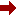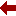#### SLIM21

 Sort Order Chronological Author Title ItemType,Author ItemType,Title Display Format Tabular Publication Bibliography Items / Page 5 10 25

Click the serial number on the left to view the details of the item.
#  AuthorTitleAccn#YearItem Type Claims
11 Smirnov, Vladimir A Feynman Integral Calculus I08286 2006 eBook
12 Zeidler, Eberhard Quantum Field Theory I: Basics in Mathematics and Physics I07526 2006 eBook
13 Shima, Hiroyuki Higher Mathematics for Physics and Engineering I07454 2010 eBook
14 Wazwaz, Abdul-Majid Partial Differential Equations and Solitary Waves Theory I07354 2009 eBook
15 Derezinski, Jan Large Coulomb Systems I06645 2006 eBook
16 Rodrigues, Waldyr A The Many Faces of Maxwell, Dirac and Einstein Equations I06614 2007 eBook
17 Economou, Eleftherios N Green's Functions in Quantum Physics I06529 2006 eBook
18 Lieb, Elliott H The Stability of Matter: From Atoms to Stars I06244 2005 eBook
19 Gerdjikov, Vladimir Integrable Hamiltonian Hierarchies I06124 2008 eBook
20 Fe??kan, Michal Bifurcation and Chaos in Discontinuous and Continuous Systems I06061 2011 eBook
 (page:2 / 12) [#111] First Page Previous Page Next Page Last Page

11.TitleFeynman Integral Calculus
PublicationBerlin, Heidelberg, Springer Berlin Heidelberg, 2006.
DescriptionX, 287 p. 52 illus : online resource
Abstract NoteThe problem of evaluating Feynman integrals over loop momenta has existed from the early days of perturbative quantum field theory. The goal of the book is to summarize those methods for evaluating Feynman integrals that have been developed over a span of more than fifty years. `Feynman Integral Calculus' characterizes the most powerful methods in a systematic way. It concentrates on the methods that have been employed recently for most sophisticated calculations and illustrates them with numerous examples, starting from very simple ones and progressing to nontrivial examples. It also shows how to choose adequate methods and combine them in a non-trivial way. This is a textbook version of the previous book (Evaluating Feynman integrals, STMP 211) of the author. Problems and solutions have been included, Appendix G has been added, more details have been presented, recent publications on evaluating Feynman integrals have been taken into account and the bibliography has been updated
ISBN,Price9783540306115
Keyword(s)1. ANALYSIS 2. Analysis (Mathematics) 3. EBOOK 4. EBOOK - SPRINGER 5. Elementary particles (Physics) 6. Elementary Particles, Quantum Field Theory 7. MATHEMATICAL ANALYSIS 8. QUANTUM FIELD THEORY 9. QUANTUM PHYSICS
Item TypeeBook
Circulation Data
 Accession# Call# Status Issued To Return Due On Physical Location I08286 On Shelf

12.TitleQuantum Field Theory I: Basics in Mathematics and Physics : A Bridge between Mathematicians and Physicists
Author(s)Zeidler, Eberhard
PublicationBerlin, Heidelberg, Springer Berlin Heidelberg, 2006.
DescriptionXXIV, 1051 p : online resource
Abstract NoteThis is the first volume of a modern introduction to quantum field theory which addresses both mathematicians and physicists ranging from advanced undergraduate students to professional scientists. The book tries to bridge the existing gap between the different languages used by mathematicians and physicists. For students of mathematics it is shown that detailed knowledge of the physical background helps to motivate the mathematical subjects and to discover interesting interrelationships between quite different mathematical topics. For students of physics, fairly advanced mathematics is presented, which is beyond the usual curriculum in physics. It is the author's goal to present the state of the art of realizing Einstein's dream of a unified theory for the four fundamental forces in the universe (gravitational, electromagnetic, strong, and weak interaction). From the reviews: "??? Quantum field theory is one of the great intellectual edifices in the history of human thought. ??? This volume differs from other books on quantum field theory in its greater emphasis on the interaction of physics with mathematics. ??? an impressive work of scholarship." (William G. Faris, SIAM Review, Vol. 50 (2), 2008) ??"??? it is a fun book for practicing quantum field theorists to browse, and it may be similarly enjoyed by mathematical colleagues. Its ultimate value may lie in encouraging students to enter this challenging interdisciplinary area of mathematics and physics. Summing Up: Recommended. Upper-division undergraduates through faculty." (M. C. Ogilvie, CHOICE, Vol. 44 (9), May, 2007)
ISBN,Price9783540347644
Keyword(s)1. ANALYSIS 2. Analysis (Mathematics) 3. EBOOK 4. EBOOK - SPRINGER 5. Elementary particles (Physics) 6. Elementary Particles, Quantum Field Theory 7. FUNCTIONAL ANALYSIS 8. MATHEMATICAL ANALYSIS 9. Mathematical Methods in Physics 10. MATHEMATICAL PHYSICS 11. PARTIAL DIFFERENTIAL EQUATIONS 12. PHYSICS 13. QUANTUM FIELD THEORY 14. Theoretical, Mathematical and Computational Physics
Item TypeeBook
Circulation Data
 Accession# Call# Status Issued To Return Due On Physical Location I07526 On Shelf

13.TitleHigher Mathematics for Physics and Engineering
Author(s)Shima, Hiroyuki;Nakayama, Tsuneyoshi
PublicationBerlin, Heidelberg, Springer Berlin Heidelberg, 2010.
DescriptionXXI, 688 p : online resource
Abstract NoteDue to the rapid expansion of the frontiers of physics and engineering, the demand for higher-level mathematics is increasing yearly. This book is designed to provide accessible knowledge of higher-level mathematics demanded in contemporary physics and engineering. Rigorous mathematical structures of important subjects in these fields are fully covered, which will be helpful for readers to become acquainted with certain abstract mathematical concepts. The selected topics are: - Real analysis, Complex analysis, Functional analysis, Lebesgue integration theory, Fourier analysis, Laplace analysis, Wavelet analysis, Differential equations, and Tensor analysis. This book is essentially self-contained, and assumes only standard undergraduate preparation such as elementary calculus and linear algebra. It is thus well suited for graduate students in physics and engineering who are interested in theoretical backgrounds of their own fields. Further, it will also be useful for mathematics students who want to understand how certain abstract concepts in mathematics are applied in a practical situation. The readers will not only acquire basic knowledge toward higher-level mathematics, but also imbibe mathematical skills necessary for contemporary studies of their own fields
ISBN,Price9783540878643
Keyword(s)1. ANALYSIS 2. Analysis (Mathematics) 3. Applications of Mathematics 4. APPLIED MATHEMATICS 5. EBOOK 6. EBOOK - SPRINGER 7. ENGINEERING MATHEMATICS 8. MATHEMATICAL ANALYSIS 9. Mathematical and Computational Engineering 10. Mathematical Methods in Physics 11. MATHEMATICAL PHYSICS 12. PHYSICS 13. Theoretical, Mathematical and Computational Physics
Item TypeeBook
Circulation Data
 Accession# Call# Status Issued To Return Due On Physical Location I07454 On Shelf

14.TitlePartial Differential Equations and Solitary Waves Theory
Author(s)Wazwaz, Abdul-Majid
PublicationBerlin, Heidelberg, Springer Berlin Heidelberg, 2009.
Description700 p. 14 illus : online resource
Abstract Note"Partial Differential Equations and Solitary Waves Theory" is a self-contained book divided into two parts: Part I is a coherent survey bringing together newly developed methods for solving PDEs. While some traditional techniques are presented, this part does not require thorough understanding of abstract theories or compact concepts. Well-selected worked examples and exercises shall guide the reader through the text. Part II provides an extensive exposition of the solitary waves theory. This part handles nonlinear evolution equations by methods such as Hirota???s bilinear method or the tanh-coth method. A self-contained treatment is presented to discuss complete integrability of a wide class of nonlinear equations. This part presents in an accessible manner a systematic presentation of solitons, multi-soliton solutions, kinks, peakons, cuspons, and compactons. While the whole book can be used as a text for advanced undergraduate and graduate students in applied mathematics, physics and engineering, Part II will be most useful for graduate students and researchers in mathematics, engineering, and other related fields. Dr. Abdul-Majid Wazwaz is a Professor of Mathematics at Saint Xavier University, Chicago, Illinois, USA
ISBN,Price9783642002519
Keyword(s)1. ANALYSIS 2. Analysis (Mathematics) 3. APPLIED MATHEMATICS 4. EBOOK 5. EBOOK - SPRINGER 6. ENGINEERING 7. ENGINEERING MATHEMATICS 8. Engineering, general 9. MATHEMATICAL ANALYSIS 10. Mathematical and Computational Engineering
Item TypeeBook
Circulation Data
 Accession# Call# Status Issued To Return Due On Physical Location I07354 On Shelf

15.TitleLarge Coulomb Systems : Lecture Notes on Mathematical Aspects of QED
Author(s)Derezinski, Jan;Siedentop, Heinz
PublicationBerlin, Heidelberg, Springer Berlin Heidelberg, 2006.
DescriptionX, 326 p : online resource
Abstract NoteA mathematically consistent formulation of relativistic quantum electrodynamics (QED) has still to be found. Nevertheless, there are several simplified effective models that successfully describe many body quantum systems and the interaction of radiation with matter. Large Coulomb Systems explores a selection of mathematical topics inspired by QED. It comprises selected, expanded and edited lectures given by international experts at a topical summer school and is intended as a high-level introduction for graduate students, postdocs and nonspecialists from related fields
ISBN,Price9783540325796
Keyword(s)1. ANALYSIS 2. Analysis (Mathematics) 3. EBOOK 4. EBOOK - SPRINGER 5. Elementary particles (Physics) 6. Elementary Particles, Quantum Field Theory 7. MATHEMATICAL ANALYSIS 8. Mathematical Methods in Physics 9. PHYSICS 10. QUANTUM FIELD THEORY
Item TypeeBook
Circulation Data
 Accession# Call# Status Issued To Return Due On Physical Location I06645 On Shelf

16.TitleThe Many Faces of Maxwell, Dirac and Einstein Equations : A Clifford Bundle Approach
Author(s)Rodrigues, Waldyr A;Oliveira, Edmundo C
PublicationBerlin, Heidelberg, Springer Berlin Heidelberg, 2007.
DescriptionXIV, 445 p : online resource
Abstract NoteThis book is a thoughtful exposition of the algebra and calculus of differential forms, the Clifford and Spin-Clifford bundles formalisms with emphasis in calculation procedures, and vistas to a formulation of some important concepts of differential geometry necessary for a deep understanding of spacetime physics. The formalism discloses the hidden geometrical nature of spinor fields. Maxwell, Dirac and Einstein fields, which were originally considered objects of a very different mathematical nature, are shown to have representatives as objects of the same mathematical nature, i.e. as sections of an appropriate Clifford bundle. This approach reveals unity in the diversity and also the many faces of the equations satisfied by those fields. Moreover, it suggests relationships which are hidden in the standard formalisms and new paths for research. Some foundational issues of relativistic field theories, in particular the one concerning the conditions for the existence of the conservation laws of energy-momentum and angular momentum in spacetime theories and many misconceptions concerning this issue is analyzed in details. The book will be useful as reference book for researchers and advanced students of theoretical physics and mathematics. Calculation procedures are illustrated by many exercises solved in detail, using the "tricks of the trade". Furthermore the readers will appreciate the comprehensive list of mathematical symbols as well as a list of acronyms and abbreviations
ISBN,Price9783540712930
Keyword(s)1. ANALYSIS 2. Analysis (Mathematics) 3. Classical and Quantum Gravitation, Relativity Theory 4. DIFFERENTIAL GEOMETRY 5. EBOOK 6. EBOOK - SPRINGER 7. GRAVITATION 8. MATHEMATICAL ANALYSIS 9. Mathematical Methods in Physics 10. PHYSICS
Item TypeeBook
Circulation Data
 Accession# Call# Status Issued To Return Due On Physical Location I06614 On Shelf

17.TitleGreen's Functions in Quantum Physics
Author(s)Economou, Eleftherios N
PublicationBerlin, Heidelberg, Springer Berlin Heidelberg, 2006.
DescriptionXVIII, 480 p : online resource
Abstract NoteThe main part of this book is devoted to the simplest kind of Green's functions, namely the solutions of linear differential equations with a -function source. It is shown that these familiar Green's functions are a powerful tool for obtaining relatively simple and general solutions of basic problems such as scattering and bound-level information. The bound-level treatment gives a clear physical understanding of "difficult" questions such as superconductivity, the Kondo effect, and, to a lesser degree, disorder-induced localization. The more advanced subject of many-body Green's functions is presented in the last part of the book
ISBN,Price9783540288411
Keyword(s)1. ANALYSIS 2. Analysis (Mathematics) 3. EBOOK 4. EBOOK - SPRINGER 5. Elementary particles (Physics) 6. Elementary Particles, Quantum Field Theory 7. MATHEMATICAL ANALYSIS 8. Mathematical Methods in Physics 9. MATHEMATICAL PHYSICS 10. Numerical and Computational Physics, Simulation 11. PHYSICS 12. QUANTUM FIELD THEORY 13. QUANTUM PHYSICS 14. Theoretical, Mathematical and Computational Physics
Item TypeeBook
Circulation Data
 Accession# Call# Status Issued To Return Due On Physical Location I06529 On Shelf

18.TitleThe Stability of Matter: From Atoms to Stars : Selecta of Elliott H. Lieb
Author(s)Lieb, Elliott H;Thirring, Walter
PublicationBerlin, Heidelberg, Springer Berlin Heidelberg, 2005.
DescriptionXVI, 932 p : online resource
Abstract NoteThis collection of papers -- starting with a brilliant article by one of the masters of the field -- gives an excellent current review of our knowledge of matter. Partially basing his own work on a variational formulation of quantum mechanics, E.H. Lieb links the difficult question of the stability of matter with important problems in functional analysis. Here the reader will find general results together with deep insights into quantum systems combined with papers on the structure of atoms and molecules, the thermodynamic limit, and stellar structures. The book is suitable as an accompanying text or recommended reading for a graduate course in quantum mechanics. In the third edition, two new sections were added: one contains papers on quantum electrodynamics, and the other on Boson systems. In this fourth edition, these topics have been further developed, extending the book by approximately 120 pages
ISBN,Price9783540270560
Keyword(s)1. ANALYSIS 2. Analysis (Mathematics) 3. CALCULUS OF VARIATIONS 4. Calculus of Variations and Optimal Control; Optimization 5. CONDENSED MATTER 6. CONDENSED MATTER PHYSICS 7. EBOOK 8. EBOOK - SPRINGER 9. Elementary particles (Physics) 10. Elementary Particles, Quantum Field Theory 11. MATHEMATICAL ANALYSIS 12. Mathematical Methods in Physics 13. PHYSICS 14. QUANTUM FIELD THEORY 15. QUANTUM PHYSICS
Item TypeeBook
Circulation Data
 Accession# Call# Status Issued To Return Due On Physical Location I06244 On Shelf

19.TitleIntegrable Hamiltonian Hierarchies : Spectral and Geometric Methods
PublicationBerlin, Heidelberg, Springer Berlin Heidelberg, 2008.
DescriptionXII, 643 p : online resource
Abstract NoteThis book presents a detailed derivation of the spectral properties of the Recursion Operators allowing one to derive all the fundamental properties of the soliton equations and to study their Hamiltonian hierarchies. Thus it is demonstrated that the inverse scattering method for solving soliton equations is a nonlinear generalization of the Fourier transform. The book brings together the spectral and the geometric approaches and as such will be useful to a wide readership: from researchers in the field of nonlinear completely integrable evolution equations to graduate and post-graduate students
ISBN,Price9783540770541
Keyword(s)1. ANALYSIS 2. Analysis (Mathematics) 3. EBOOK 4. EBOOK - SPRINGER 5. GEOMETRY 6. MATHEMATICAL ANALYSIS 7. Mathematical Methods in Physics 8. MATHEMATICAL PHYSICS 9. PHYSICS 10. Physics, general 11. Theoretical, Mathematical and Computational Physics
Item TypeeBook
Circulation Data
 Accession# Call# Status Issued To Return Due On Physical Location I06124 On Shelf

20.TitleBifurcation and Chaos in Discontinuous and Continuous Systems
Author(s)Fe??kan, Michal
PublicationBerlin, Heidelberg, Springer Berlin Heidelberg, 2011.
DescriptionXII, 378 p : online resource
Abstract Note"Bifurcation and Chaos in Discontinuous and Continuous Systems" provides rigorous mathematical functional-analytical tools for handling chaotic bifurcations along with precise and complete proofs together with concrete applications presented by many stimulating and illustrating examples. A broad variety of nonlinear problems are studied involving difference equations, ordinary and partial differential equations, differential equations with impulses, piecewise smooth differential equations, differential and difference inclusions, and differential equations on infinite lattices as well. This book is intended for mathematicians, physicists, theoretically inclined engineers and postgraduate students either studying oscillations of nonlinear mechanical systems or investigating vibrations of strings and beams, and electrical circuits by applying the modern theory of bifurcation methods in dynamical systems. Dr. Michal Fe??kan is a Professor at the Department of Mathematical Analysis and Numerical Mathematics on the Faculty of Mathematics, Physics and Informatics at the Comenius University in Bratislava, Slovakia. He is working on nonlinear functional analysis, bifurcation theory and dynamical systems with applications to mechanics and vibrations
ISBN,Price9783642182693
Keyword(s)1. ANALYSIS 2. Analysis (Mathematics) 3. CLASSICAL MECHANICS 4. DYNAMICAL SYSTEMS 5. Dynamical Systems and Ergodic Theory 6. DYNAMICS 7. EBOOK 8. EBOOK - SPRINGER 9. ERGODIC THEORY 10. MATHEMATICAL ANALYSIS 11. MATHEMATICAL PHYSICS 12. MECHANICS 13. Theoretical, Mathematical and Computational Physics 14. VIBRATION 15. Vibration, Dynamical Systems, Control
Item TypeeBook